# A circuit is made of two 1.4 volt batteries and three light bulbs as shown in...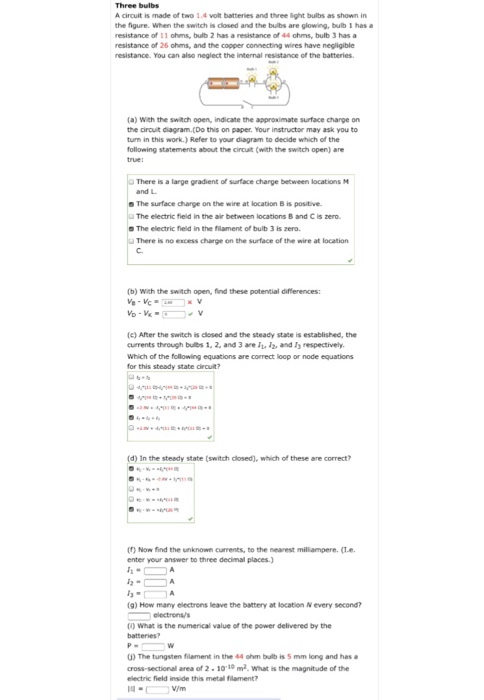A circuit is made of two 1.4 volt batteries and three light bulbs as shown in the figure. When the switch is closed and the buibs are glowing, bulb I hasa resistance of 11 ohms, bulb 2 has a resistance of 44 ohms, bulb 3 has a resistance of 26 ohms, and the copper connecting wires have negligible resistance. You can also neglect the internal resistance of the batteries (a) With the swtch open, indicate the approximate surface charge on the cirouit ciagram.(Do this on paper. Your instructor may ask you to turn in this work.) Refer to your diagram to decide which of the following statements about the circuit (with the switch open) are There is a large gradient of surface charge between locations M and L The surface charge on the wire at location B is positive. The electric field in the air between locations B and C is zero. The electric field in the filament of bulb 3 is zero. There is no excess charge on the surface of the wire at location C. (b) With the switch open, find these potential differences: (c) After the switch is closed and the steady state is established, the currents through bubs 1, 2, and 3 are I. Iz, and Jy respectively. Which of the followling equations are correct loop or node equations for this steady state circuit? (d) in the steedy state (switch closed). which of these are correct? T) Now find the unknown currents, to the nearest milliampere. (T.e. enter your answer to three decimmal places (9) How many electrons leave the battery at location N every second? What is the numerical value of the power delivered by the U) The tungsten filament in the 44 ohm bulb is 5 mm long and has a cross-sectional area of 2.101 m2. What is the magnitude of the electric field inside this metal filament?

(b) When the switch is open the current through the circuit is zero. So, total voltage dropped across the open circuited switch.

VB - V C = V = 1.4+1.4

VB - V C = 2.8 Volts

(f) You can use resistances to solve this problem

I1 is the master current. It will equal the master voltage from the batteries, divided by the total circuit equivalent resistance.

To get the total circuit equivalent resistance, we first combine R2 and R3 in parallel to get R23. Then we combine R23 in series with R1.

Rnet = R1 + R2*R3/(R2 + R3)

Note: use the "product over sum" shortcut with caution. This is only applicable for two branches of resistances.

I1 = Vbat / Rnet
I1 = 2 * Vbat/Rnet
I1 = 2*Vbat / (R1 + R2*R3/(R2 + R3))

I1 = 2*1.4/ (11 + [(44*26) / (44 + 26) ] )

I1 = 0.1024 A

Now, divide this current up, according to the CONDUCTANCE fractions of both paths. The conductances are the reciprocals of resistances. The conductance fractions are the fractions of the total conductance.

Total conductance:
1/R2 + 1/R3

Conductance fraction of path through R2:
Y2 = (1/R2)/(1/R2 + 1/R3)
Y2 = 0.3714

Conductance fraction of path through R3:
Y3 = (1/R3)/(1/R2 + 1/R3)
Y3 = 0.6285

I2 = I1*Y2 = 0.1024*0.3714 = 0.038
I3 = I1*Y3 = 0.1024*0.6285 = 0.064

Results:
I1 = 0.102 A
I2 = 0.038 A
I3 = 0.064 A

(g) The current flowing at location N, is I1. It is flowing in to the battery, thus electrons are exiting the battery. We need to translate from amperes per second, into elementary charge units per second, since the charge of 1 electron is 1 elementary charge unit. 1 elementary charge unit is 1.602 * 10-19 Coulombs. And the Ampere is 1 Coulomb per second. Believe it or not, the ampere came first in the original definitions.

Divide by the elementary charge unit, in Coulombs
0.102 A / 1.602 * 10-19 C  = 6.367*1017 electrons/second

(i) Multiply the master current and voltage to get the total power.
P = I1*Vbat = 0.102 * 2.8

P = 0.2856 Watts

( j ) Electric field is voltage divided length, assuming that it is uniform. In a wire such as this, we might as well assume it is uniform. The cross sectional area doesn't really matter, all that does is assist us in confirming that it is 44 ohms.

E = V/L
V = I2*R2
E = I2*R2/L
E = (0.038 A)*(44 ohms)/(0.005 m)

|E| = 334.4 V/m

#### Earn Coin

Coins can be redeemed for fabulous gifts.

Similar Homework Help Questions
• ### Three bulbs A circuit is made of two 1.7 volt batteries and three light bulbs as...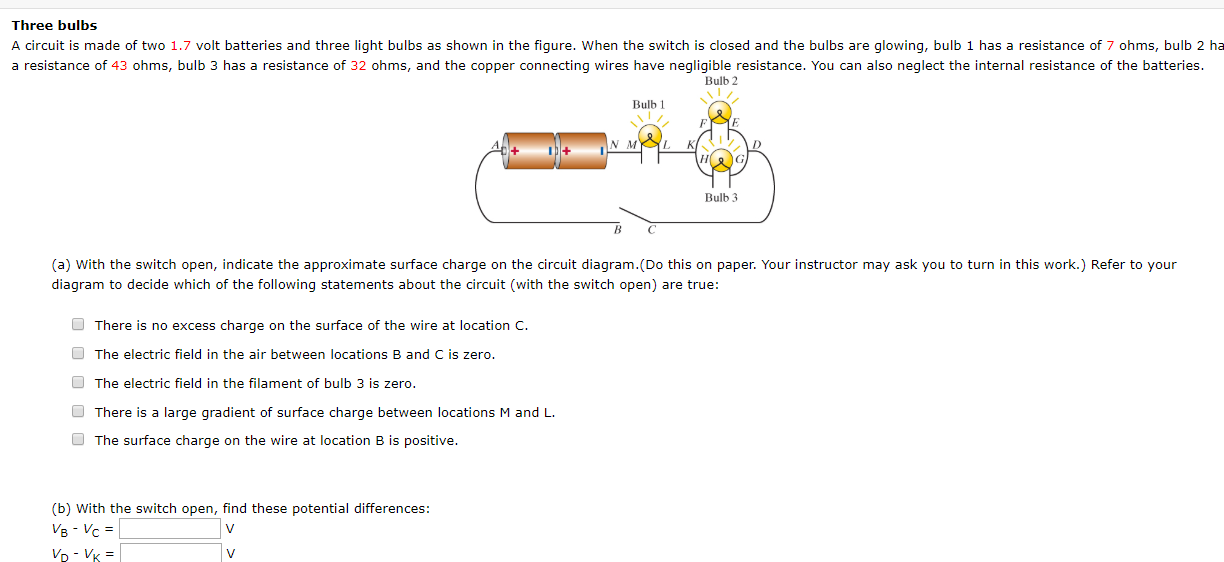Three bulbs A circuit is made of two 1.7 volt batteries and three light bulbs as shown in the figure. When the switch is closed and the bulbs are glowing, bulb 1 has a resistance of 7 ohms, bulb 2 ha a resistance of 43 ohms, bulb 3 has a resistance of 32 ohms, and the copper connecting wires have negligible resistance. You can also neglect the internal resistance of the batteries Bulb 2 Bulb 1 Bulb 3 (a) With...

• ### Two batteries, a capacitor, and two light bulbs are connected by wires. The batteries have a...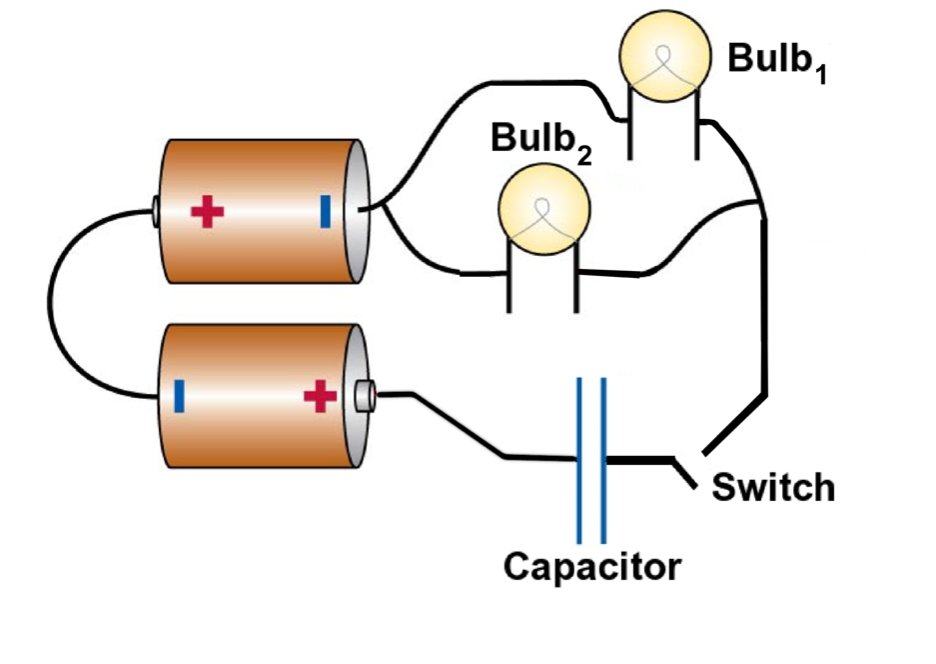Two batteries, a capacitor, and two light bulbs are connected by wires. The batteries have a potential difference emf, the capacitor has capacitance C, and the bulbs have resistance R. Initially the capacitor is uncharged and the switch has been closed. A)Write down the three loop rules and the node rule for this circuit and label the currents on the picture. B) Find the initial currents running through each bulb. C)What is the current running through each bulb a long...

• ### Three identical light bulbs are connected to two batteries as shown in the diagram above.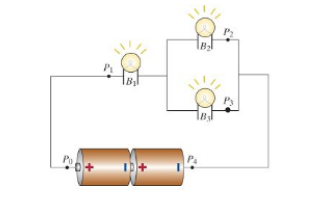Three identical light bulbs are connected to two batteries as shown in the diagram above.To start the analysis of this circuit you must write energy conservation (loop) equations. Each equation must involve around-trip path that begins and ends at the same location. Each segment of the path should go through a wire, a bulb, or a battery(not through the air). How many valid energy conservation (loop)equations is it possible to write for this circuit?---Select---012345Which of the following equations are valid...

• ### Problem 3:) A light bulb is connected in the circuit shown in the figure with the...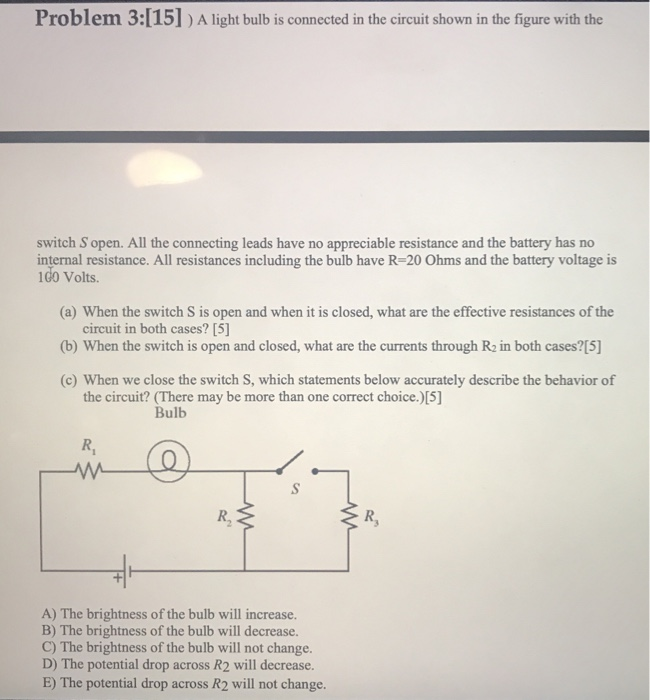Problem 3:) A light bulb is connected in the circuit shown in the figure with the switch S open. All the connecting leads have no appreciable resistance and the battery has no internal resistance. All resistances including the bulb have R=20 Ohms and the battery voltage is 1do Volts. (a) When the switch S is open and when it is closed, what are the effective resistances of the circuit in both cases?  (b) When the switch is open and...

• ### - points MI4 13.3034 Three bulbs The figure is a top view of a circuit containing...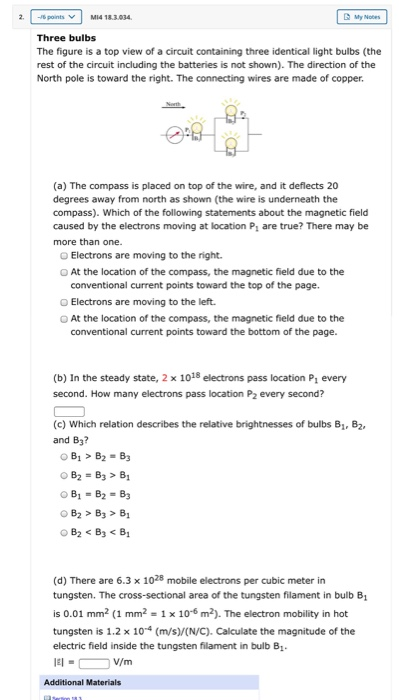- points MI4 13.3034 Three bulbs The figure is a top view of a circuit containing three identical light bulbs (the rest of the circuit including the batteries is not shown). The direction of the North pole is toward the right. The connecting wires are made of copper. 3: (a) The compass is placed on top of the wire, and it deflects 20 degrees away from north as shown (the wire is underneath the compass). Which of the following statements...

• ### In the circuit shown in the diagram below, two thick wires connect a 1.4 volt battery...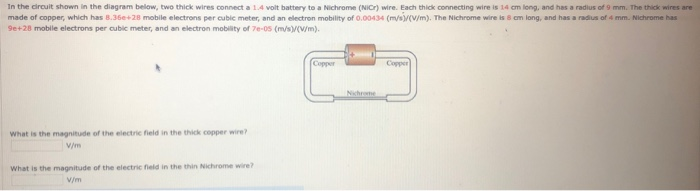In the circuit shown in the diagram below, two thick wires connect a 1.4 volt battery to a Nichrome (NIC) wire. Each thick connecting wire is 14 cm long, and has a radius of 9 mm. The thick wires are made of copper, which has 8.35+28 mobile electrons per cubic meter, and an electron mobility of 0.00434 (m (V/m). The Nichrome wire is 8 cm long, and has a radius of 4 mm. Nichrome has 9e+28 mobile electrons per cubic...

• ### P48 In the circuit shown in Figure 18.110, the two thick wires and the thin wire are made of Nich...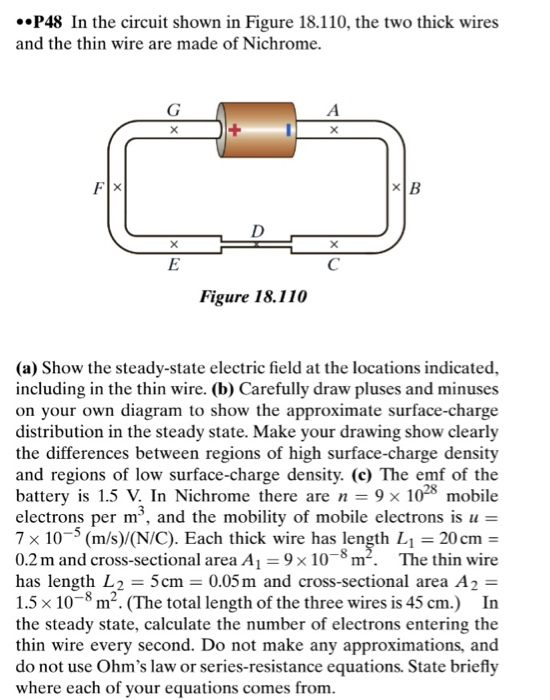Please answer P48 P48 In the circuit shown in Figure 18.110, the two thick wires and the thin wire are made of Nichrome. Figure 18.110 (a) Show the steady-state electric field at the locations indicated, including in the thin wire. (b) Carefully draw pluses and minuses on your own diagram to show the approximate surface-charge distribution in the steady state. Make your drawing show clearly the differences between regions of high surface-charge density and regions of low surface-charge density. (c)...

• ### Basic aspects of electron current flow in circuits (Please help and you will get lifesaver)...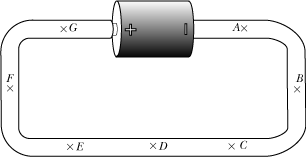A steady-state current flows through the Nichrome wire in thecircuit shown in Figure 18.64.Figure 18.64Before attempting to answer these question, draw a copyof this diagram. All of the locations indicated by letters areinside the wire.(a) On your diagram, show the electric field at the locationsindicated, paying attention to relative magnitude.(b) Carefully draw pluses and minuses on your diagram to show theapproximate surface charge distribution that produces the electricfield you drew. Make yourdrawing show clearly the differencesbetween regions of high surface...

• ### ON CAPAC Transient RL Circuit Consider the circuit shown in the figure. The resistance of the...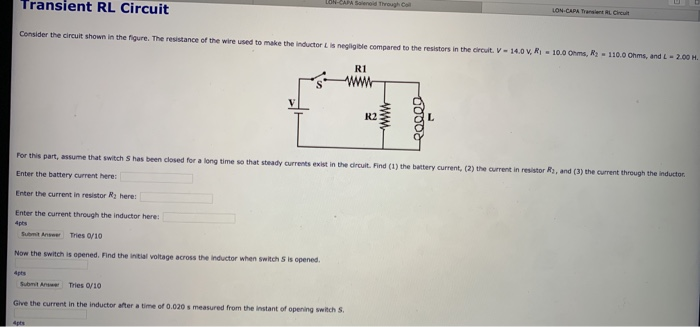ON CAPAC Transient RL Circuit Consider the circuit shown in the figure. The resistance of the wire used to make the inductor is negligible compared to the resistors in the circuit. V-140 V, R; -10.Ohms, Ry - 1100 Ohms, and L-200 H RI WWW For this part, assume that switch S has been closed for a long time so that steady currents exist in the circuit. Find (1) the battery current, (2) the current in resistor Ry, and (3) the...

• ### A common demonstration of inductance employs a circuit such as the one shown in the figure...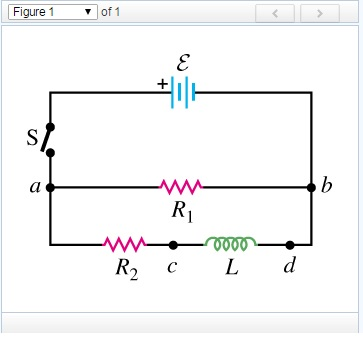A common demonstration of inductance employs a circuit such as the one shown in the figure (Figure 1) . Switch S is closed, and the light bulb (represented by resistance R1) just barely glows. After a period of time, switch S is opened, and the bulb lights up brightly for a short period of time. To understand this effect, think of an inductor as a device that imparts an "inertia" to the current, preventing a discontinuous change in the current...